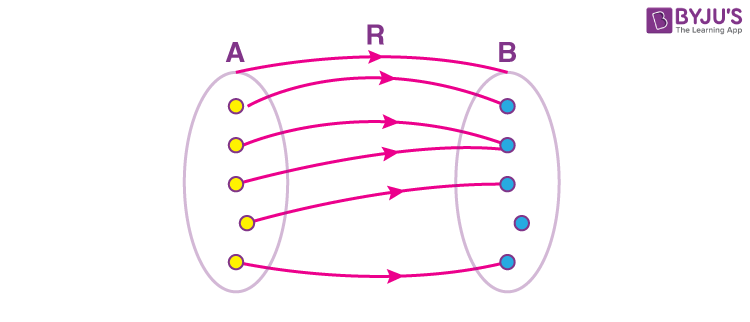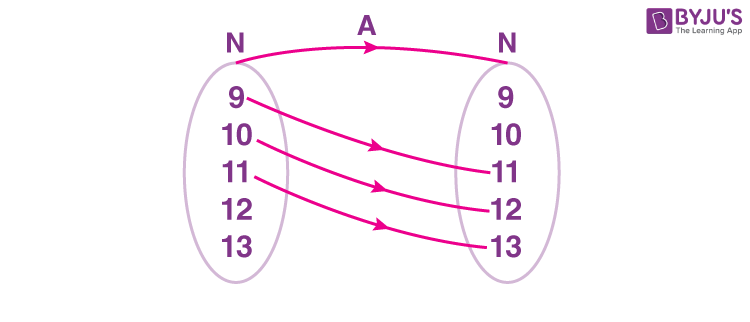# Domain And Range Of A Relation

To know Domain and Range of a relation, let us understand what relations are.

Relations:

To understand what relations are, we should first understand what Cartesian product is. A Cartesian productof two sets A and B is a collection of all the ordered pairs (a,b) such that a ∈ A and b ∈ B . A relation is a subset of a Cartesian product. Basically, a relation is a rule that “relates” an element from one set to an element from another set.

Definition: If there are two non-empty sets A and B , a relation R  is defined as a subset of the Cartesian product A × B . This subset is the result of the “relation” defined between the elements of the first and the second set.

R = {(x,y):x ∈ A and y ∈ B}In figure 1, there is a relation from set A to B . All the circular dots are “related” to the square dots with R . A relation may have finite or infinite ordered pairs. If we take a relation from set A to A , it is commonly referred as ‘relation on A’. The maximum number of relations that can be defined from set A (having m elements) to B (having n elements) is equal to

$$\begin{array}{l} 2^{mn}\end{array}$$
..

## Domain and Range of a Relation:

Now, it’s clear that relation just like a Cartesian product will have ordered pairs. In these ordered pairs, second element is known as the image of the first element and the first element is known as the preimage of the second element.

This means that among the two sets, one will have all the preimages and the other will have all the images. The set which contains all the first elements of all the ordered pairs of relation  R is known as the domain of the relation. The domain set may or may not be equal to the set A as shown in figure 1. The set which contains all the second elements, on the other hand, is known as the range of the relation. Now, here lies a catch. The second set  B can be equal to the range of the relation or it can be bigger than that. This is because there can be elements in B which are not related to any element in set A. That’s why the whole set B  is called by a different name i.e.

Codomain of the relation. It is very clear that

Range ⊆ Codomain

Relations can also be represented in the way in which we represent sets i.e. Roster method or Set-builder method. Another better understandable (visually) representation is given using the arrow diagrams (Fig. 1).

For e.g. Let us define a set N = {9,10,11,12,13}. Now, let us define a relation A from N  to N such that in the ordered pair (x,y)  in A , y is two more than x. This can be represented in three different ways:

• Set-builder method:A = { (x,y):y = x + 2,x , y ∈ N}
• Roster method: A = { (9,11) , (10,12), (11,13)}
• Arrow diagram:Now, domain of  A is not N. It is equal to {9,10,11}. This is because, for other values from N, the output doesn’t lie in set N. Also, the codomain of A is N. But the range of A  is {11,12,13}, that is a subset of the codomain.

## Video Lesson

### Variation in Range with Domain## Recommended Videos

### Relations and Types of Relations### Functions – Introduction, Co-domain and Range### Functions and Types of Functions### Range of Functions – Concepts & QuestionsRelations in mathematics are important because they help explain one of the most important concepts in mathematics. To learn more on domain and range of a relation, download BYJU’S-The Learning App.